Custom SearchPOLAR COORDINATES So far we have located a point in a plane by giving the distances of the point from two perpendicular lines. We can define the location of a point equally well by noting its distance and bearing. This method is commonly used aboard ship to show the position of another ship or target. Thus, 3 miles at 35 locates the position of a ship relative to the course of the ship making the reading. We can use this method to develop curves and bring out their properties. Assume a fixed direction OX and a fixed point O on the line in figure 2-19. The position of any point, P, is fully determined if we know the directed distance from O to P and the angle that the line OP makes with reference line OX. The line OP is called the radius vector and the angle POX is called the polar angle. The radius vector is denoted by Q, while 0 denotes the polar angle. Point O is the pole or origin. As in conventional trigonometry, the polar angle is positive when measured counterclockwise and negative when measured clockwise. However, unlike the convention established in trigonometry, the radius vector for polar coordinates is positive only when it is laid off on the terminal side of the angle. When the radius vector is laid off on the terminal side of the ray produced beyond the pole (the given angle plus 180'), nates. a negative value is assigned the radius vector. For this reason, more than one equation may be used in polar coordinates to describe a given locus. It is sufficient that you remember that the radius vector can be negative. In this course, however, the radius vector, Q, will always be positive.Figure 2-19.-Defining the polar coordi TRANSFORMATION FROM CARTESIAN TO POLAR COORDINATES At times you will find working with the equation of a curve in polar coordinates will be easier than working in Cartesian coordinates. Therefore, you need to know how to change from one system to the other. Sometimes both forms are useful, for some properties of the curve may be more apparent from one form of the equation. We can make transformations by applying the following equations, which can be derived from figure 2-20: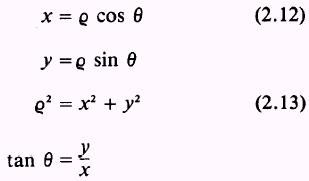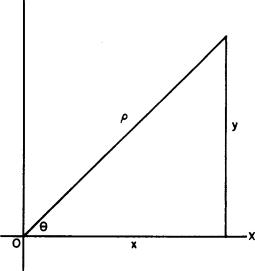Figure 2-20.-Cartesian and polar relationship.   EXAMPLE: Change the equation y=x2 from rectangular to polar coordinates. SOL UTION: Substitute Q cos e for x and Q sin 0 for y so that we haveor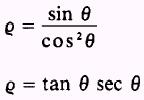EXAMPLE: Express the equation of the following circle with its center at (a,0) and with radius a, as shown in figure 2-21, in polar coordinates:SOLUTION: First, expanding this equation gives us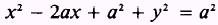Rearranging terms, we have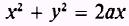The use of equation (2.13) gives us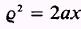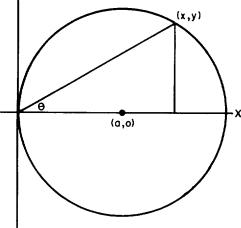Figure 2-21.-Circle with center (a,0). and applying the value of x given by equation (2.12), results in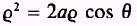Dividing both sides by Q, we have the equation of a circle with its center at (a,0) and radius a in polar coordinates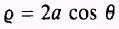Integrated Publishing, Inc. - A (SDVOSB) Service Disabled Veteran Owned Small Business